Test: Building With Bricks - 4

# Test: Building With Bricks - 4

Test Description

## 10 Questions MCQ Test Mathematics for Class 4: NCERT | Test: Building With Bricks - 4

Test: Building With Bricks - 4 for Class 4 2022 is part of Mathematics for Class 4: NCERT preparation. The Test: Building With Bricks - 4 questions and answers have been prepared according to the Class 4 exam syllabus.The Test: Building With Bricks - 4 MCQs are made for Class 4 2022 Exam. Find important definitions, questions, notes, meanings, examples, exercises, MCQs and online tests for Test: Building With Bricks - 4 below.
Solutions of Test: Building With Bricks - 4 questions in English are available as part of our Mathematics for Class 4: NCERT for Class 4 & Test: Building With Bricks - 4 solutions in Hindi for Mathematics for Class 4: NCERT course. Download more important topics, notes, lectures and mock test series for Class 4 Exam by signing up for free. Attempt Test: Building With Bricks - 4 | 10 questions in 20 minutes | Mock test for Class 4 preparation | Free important questions MCQ to study Mathematics for Class 4: NCERT for Class 4 Exam | Download free PDF with solutions
 1 Crore+ students have signed up on EduRev. Have you?
Test: Building With Bricks - 4 - Question 1

### Line m and four points are shown on the grid. Which two points appear to lie on the same line that is similar to line m?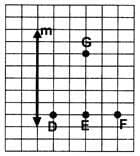Detailed Solution for Test: Building With Bricks - 4 - Question 1

Points G and E appear to lie on the same line that is similar to line m.

Test: Building With Bricks - 4 - Question 2

### The cube shown here was cut into three pieces- Which of the following groups CANNOT be the three pieces of the cube?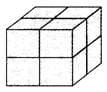Detailed Solution for Test: Building With Bricks - 4 - Question 2

Among all the given options, option [C] cannot be the three pieces of the cube.

Test: Building With Bricks - 4 - Question 3

### The perimeters of square P and rectangle Q are equal. Find the breadth of rectangle Q.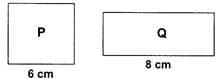Detailed Solution for Test: Building With Bricks - 4 - Question 3

Perimeter of square P = 4 ×side = 4×6=24cm Perimeter of rectangle Q = 2 (Length + Breadth)
= 2 (8 + Breadth)

We are given Perimeter of P = Perimeter of Q ⇒24 = 2(8 + Breadth)

⇒2 × Breadth = 8

⇒Breadth = 4 cm

Test: Building With Bricks - 4 - Question 4

Veer plotted 3 points on a grid line. The three points together make a ______.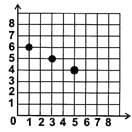Detailed Solution for Test: Building With Bricks - 4 - Question 4

The three points will form a straight line.

Test: Building With Bricks - 4 - Question 5

How many cubes are needed to make the given solid?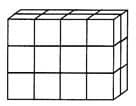Detailed Solution for Test: Building With Bricks - 4 - Question 5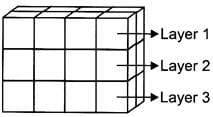Number of cubes in layer 1=8  Number of cubes in layer 2=8 Number of cubes in layer  3=8 Total number of cubes = 24

Test: Building With Bricks - 4 - Question 6

Find the perimeter of the shaded part of the given Figure.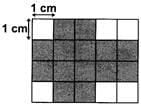Detailed Solution for Test: Building With Bricks - 4 - Question 6

Counting the sides of the shaded squares while keeping in mind that common sides are not counted twice.

Test: Building With Bricks - 4 - Question 7

Which of the following figures have a line of symmetry?

Detailed Solution for Test: Building With Bricks - 4 - Question 7

Figure in option [D] have a line symmetry.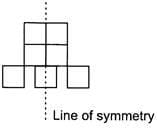A line of symmetry is a line that cuts a shape exactly in half.

This means that if you were to fold the shape along the line, both halves would match exactly. Equally, if you were to place a mirror along the line, the shape would remain unchanged.

Test: Building With Bricks - 4 - Question 8

Study the given graph. Which of the given figures show pair of intersecting lines?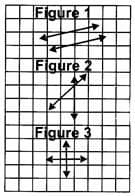Detailed Solution for Test: Building With Bricks - 4 - Question 8

Two or more lines are said to be intersecting if they meet each other at a point. Here, Figure 2 and Figure 3 shows intersecting lines.

Test: Building With Bricks - 4 - Question 9

Find the perimeter of the given figure.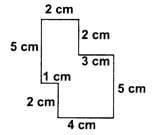Detailed Solution for Test: Building With Bricks - 4 - Question 9

Perimeter of the given figure = 5 + 2 + 2 + 3 + 5 + 4 + 2 + 1 = 24cm

Test: Building With Bricks - 4 - Question 10

Which of the following figures consists of exactly 6 faces?

Detailed Solution for Test: Building With Bricks - 4 - Question 10

Figure in option [B] has exactly 6 faces.

## Mathematics for Class 4: NCERT

24 videos|44 docs|32 tests
 Use Code STAYHOME200 and get INR 200 additional OFF Use Coupon Code
Information about Test: Building With Bricks - 4 Page
In this test you can find the Exam questions for Test: Building With Bricks - 4 solved & explained in the simplest way possible. Besides giving Questions and answers for Test: Building With Bricks - 4, EduRev gives you an ample number of Online tests for practice

## Mathematics for Class 4: NCERT

24 videos|44 docs|32 tests